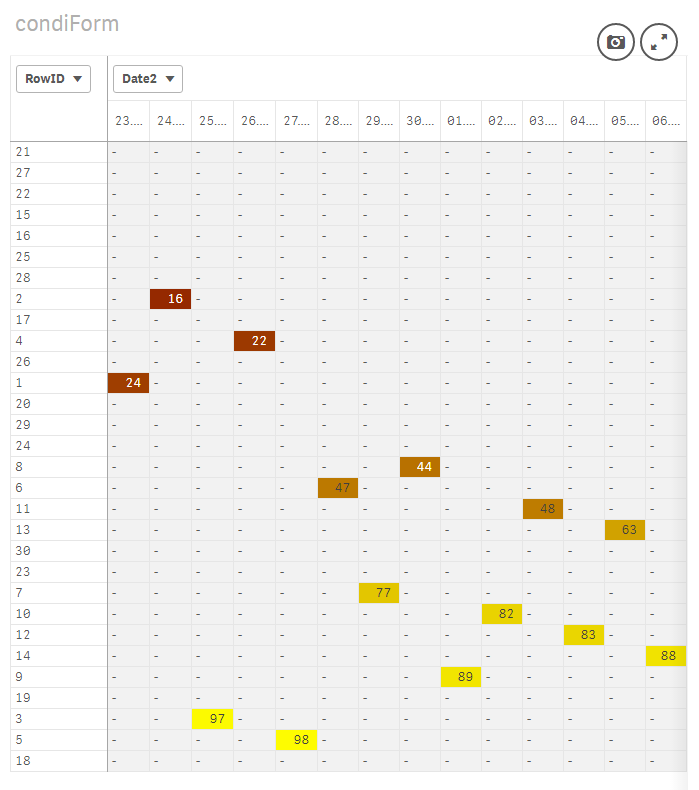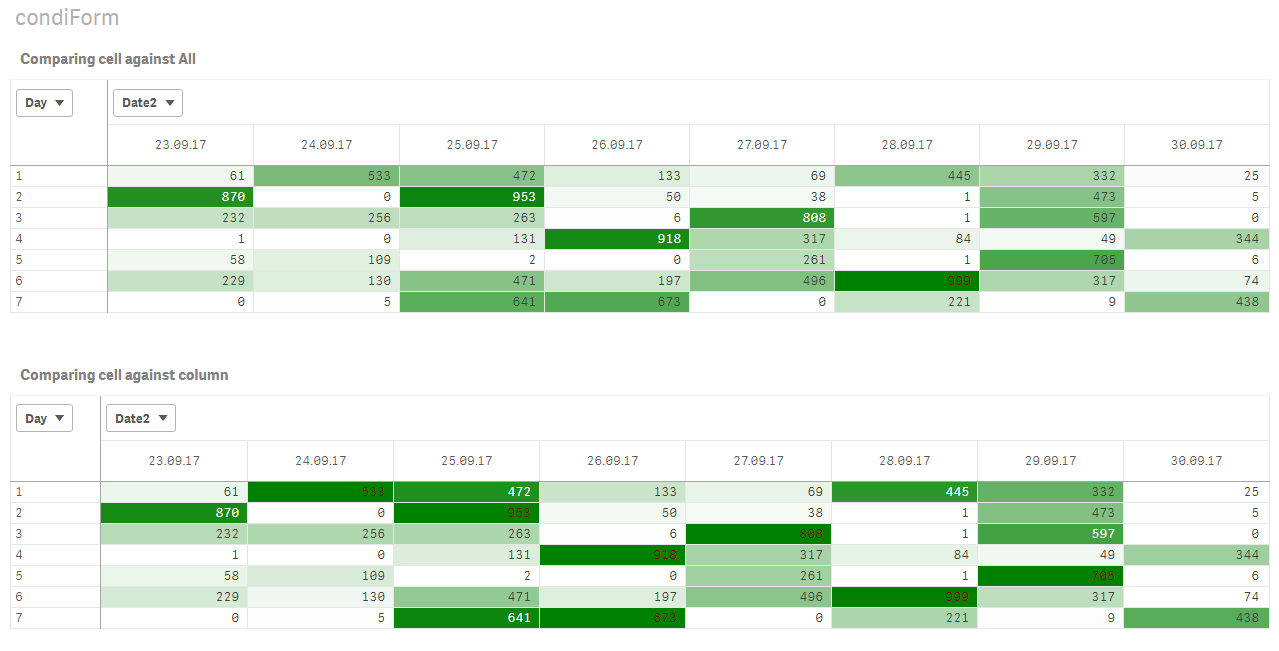Announcements
The way to achieve your own success is the willingness to help somebody else. Go for it!
cancel
Showing results for
Did you mean:Anonymous
Not applicable

## Qlik Sense conditional coloring based on highest values in column

Hello,

I would like to highlight the highest values in the columns of my pivot. (gradient from largest to smallest) How do i do that?8 RepliesSpecialist

Hi Mikko,

Let's say your measure is called Sum(MeasureValue), and those numbers you have there from 1 to 29, let's call them RowID. And that you want the lowest in Red and the highest in Yellow,

Then you could use this formula on the colour expression (background or text)

=Colormix1(

(sum(MeasureValue)-\$(=min(aggr( sum(MeasureValue), RowID))))/

\$(=(max(aggr( sum(MeasureValue),RowID))-min(aggr( sum(MeasureValue),RowID)))),Red(),Yellow()

)

Cheers,

LuisSpecialist

It'll look something like this:I hope this helps,

Cheers,

LuisSpecialist

Hi Mikko,

Have you been able to check it out?

LuisAnonymous
Not applicable
Author

Hello,

not working. the measure value is an aggregated one and the row is day(date).

And as you see i have multiple values in one column and i want to highlight the values within the columns (high to low in column)

thanksSpecialist

Hi,

Can you share sample data and your aggregation formula to see if I can reproduce?

LuisSpecialist

Hi Mikko, which one?Specialist

Hi Mikko,

Please let me know if you're still interested in solving this one. If so, please share sample data and the expressions you're using to calculate dimensions and measures so I can adapt the color expression

This is the expression I used for the second option (Comparing cell against column)

=Colormix1(

((sum(MeasureValue)-min(TOTAL <RowID> aggr(sum(MeasureValue),RowID,Date2)))

/ (max(TOTAL <RowID> aggr(sum(MeasureValue),RowID,Date2))

-min(TOTAL <RowID> aggr(sum(MeasureValue),RowID,Date2)))),White(),Green()

)

I hope this helps,

Cheers,

LuisAnonymous
Not applicable
Author

sorry, been busy. I will try out the latter option and let you know how it goes.Tags
Community Browser